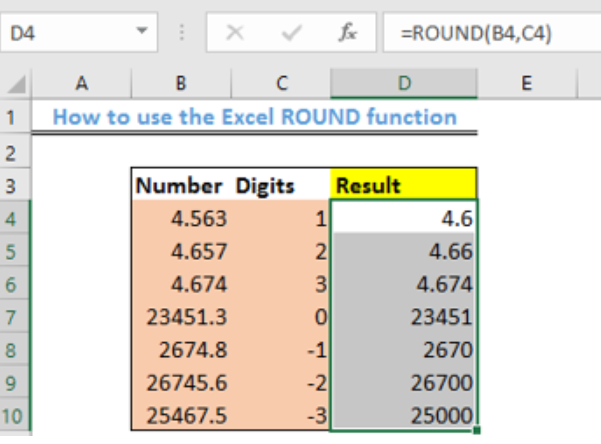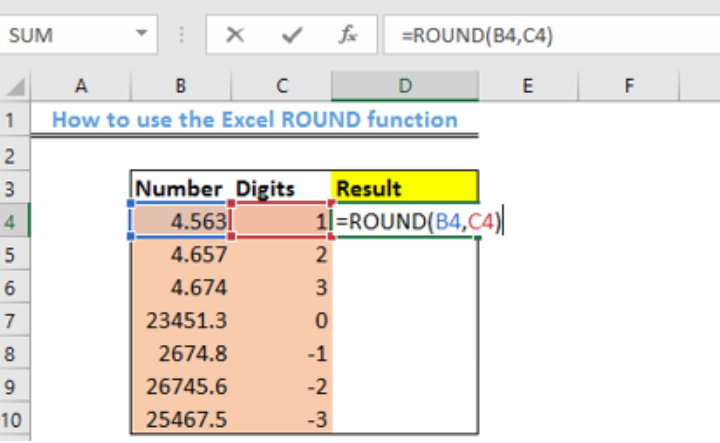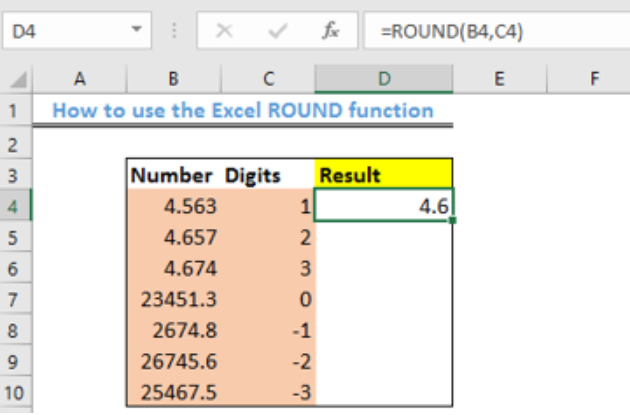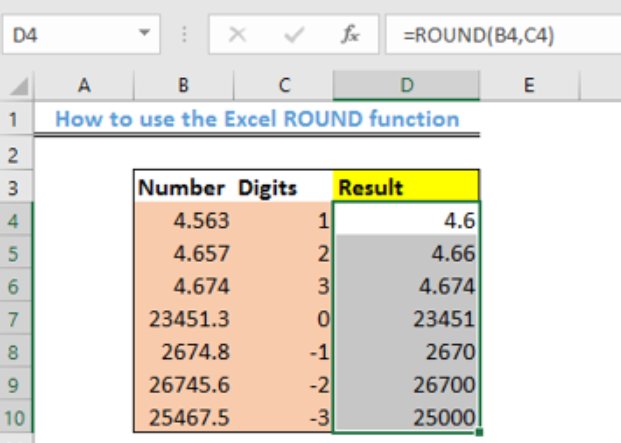Get instant live expert help with Excel or Google Sheets“My Excelchat expert helped me in less than 20 minutes, saving me what would have been 5 hours of work!”

#### Post your problem and you'll get expert help in seconds

Your message must be at least 40 characters
Our professional experts are available now. Your privacy is guaranteed.

# Excel ROUND Function

We use the Excel ROUND function to round digits of a specific number to the right or left of the decimal point. The simple steps below will walk through the process.Figure 1- Final Result with Excel ROUND function

## General Formula

`=ROUND (number, num_digits)`

• Number – the number we want to round.
• Num_digits – the number of digits we want to round the NUMBER to.

## Formula

`=ROUND(B4,C4)`

## Setting up the Data

We will apply the ROUND function to a specific set of numbers, rounding it off to an assigned digit.

• We will place the list of numbers in Cell B4 to Cell B10
• Column C will contain the list of digits we wish to round the numbers to
• Column D will hold the result as shown in figure 2.Figure 2 – Setting up the Data

## Applying the ROUND Function

• We will click on Cell D4
• We will insert the formula below into Cell D4
`=ROUND(B4,C4)`Figure 3- The Excel ROUND function

• We will press the enter keyFigure 4- Applying the ROUND function

• Using the fill handle, we will copy the formula into the remaining cellsFigure 5- How to use the ROUND function

## Explanation

The ROUND function performs the standard rounding up process. This function rounds up numbers down when the digit is between 1 and 4 and rounds up numbers when it is between 5 and 9. The ROUND function returns with the exact number of digits you specified either to the right or left side of the decimal point.

• If num_digits > 0, the number will be approximated to the stated number of decimal places to the right of the decimal point.
• If num_digits < 0, the number is rounded to the left of the decimal point (i.e., to the nearest 10, 100, 1000, etc.).
• If num_digits = 0, the number is approximated to the closest figure.

## Instant Connection to an Expert through our Excelchat Service

Most of the time, the problem you will need to solve will be more complex than a simple application of a formula or function. If you want to save hours of research and frustration, try our live Excelchat service! Our Excel Experts are available 24/7 to answer any Excel question you may have. We guarantee a connection within 30 seconds and a customized solution within 20 minutes.

### Did this post not answer your question? Get a solution from connecting with the expert.Another blog reader asked this question today on Excelchat:## Subscribe to Excelchat.coAnother blog reader asked this question today on Excelchat: